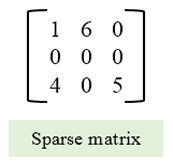## C program to check sparse matrix

Write a C program to read elements in a matrix and check whether matrix is Sparse matrix or not. C program for determining sparse matrix. How to check sparse matrix in C. Logic to check sparse matrix in C programming.

#### What is Sparse matrix?

Sparse matrix is a special matrix with most of its elements are zero. We can also assume that if (m * n) / 2 elements are zero then it is a sparse matrix.

#### Logic to check sparse matrix

To check whether a matrix is sparse matrix we only need to check the total number of elements that are equal to zero. The matrix is sparse matrix if T ≥ ((m * n) / 2 ); where T defines total number of zero elements.#### Program to check sparse matrix

```

/**
* C program to check sparse matrix
*/

#include <stdio.h>
#define SIZE 3

int main()
{
int A[SIZE][SIZE];
int row, col, total=0;

/* Input elements in matrix from user */
printf("Enter elements in matrix of size 3x3: \n");
for(row=0; row<SIZE; row++)
{
for(col=0; col<SIZE; col++)
{
scanf("%d", &A[row][col]);
}
}

/* Count total number of zero elements in the matrix */
for(row=0; row<SIZE; row++)
{
for(col=0; col<SIZE; col++)
{
/* If the current element is zero */
if(A[row][col] == 0)
{
total++;
}
}
}

if(total >= (row * col)/2)
{
printf("\nThe given matrix is a Sparse matrix.");
}
else
{
printf("\nThe given matrix is not Sparse matrix.");
}

return 0;
}

```

#### Program to check sparse matrix

```
/**
* C program to check sparse matrix
*/

#include <stdio.h>

int main()
{
int A;
int row, col, total=0,n;
printf("Enter size of matrix:");
scanf("%d",&n);

/* Input elements in matrix from user */
printf("Enter elements in matrix of size 3x3: \n");
for(row=0; row<n; row++)
{
for(col=0; col<n; col++)
{
scanf("%d", &A[row][col]);
}
}

/* Count total number of zero elements in the matrix */
for(row=0; row<n; row++)
{
for(col=0; col<n; col++)
{
/* If the current element is zero */
if(A[row][col] == 0)
{
total++;
}
}
}

if(total >= (row * col)/2)
{
printf("\nThe given matrix is a Sparse matrix.");
}
else
{
printf("\nThe given matrix is not Sparse matrix.");
}

return 0;
}

```

##### Output: Enter size of matrix:3 Enter elements in matrix of size 3x3: ```1 0 0 4 5 0 0 0 0 ``` The given matrix is a Sparse matrix. Process returned 0 (0x0) execution time : 32.152 s Enter size of matrix:3 Enter elements in matrix of size 3x3: ```3 2 1 0 0 0 2 0 0 ``` The given matrix is a Sparse matrix. Process returned 0 (0x0) execution time : 20.936 s Enter size of matrix:3 Enter elements in matrix of size 3x3: ```1 2 3 4 5 0 7 0 0 ``` The given matrix is not Sparse matrix. Process returned 0 (0x0) execution time : 13.607 s